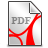# Geometries of O

Summary

Geometries of O adhere to Ockham's principle of simplest possible ontology: the only individuals are points, there are no straight lines, circles, angles etc. , just as it was was laid down by Tarski in the 1920s, when he put forward a set of axioms that only contain two relations, quaternary congruence and ternary betweenness. However, relations are not as intuitive as functions when constructions are concerned. Therefore the planar geometries of O contain only functions and no relations to start with. Essentially three quaternary functions occur: appension for line-joining of two pairs of points, linisection representing intersection of straight lines and circulation corresponding to intersection of circles. Functions are strictly defined by composition of given ones only.

Both, Euclidean and Lobachevskyan planar geometries are developed using a precise notation for object-language  and metalanguage, that allows for a very broad area of mathematical systems up to theory of types. Some astonishing results are obtained, among them:

(A)  Based on a special triangle construction Euclidean planar geometry can start with a less powerful ontological basis than Lobachevskyan geometry.

(B)  Usual Lobachevskyan planar geometry is not complete, there are nonstandard planar Lobachevskyan geometries. One needs a further axiom, the 'smallest' system is produced by the proto-octomidial-axiom.

(C)  Real numbers can be  abandoned in connection with planar geometry. A very promising conjecture is put forward, stating that the Euclidean Klein-model of Lobachevskyan planar geometry does not contain all points of the constructive Euclidean unit-circle.GeoO1.0.pdf (2.08MB)GeoO1.0.pdf (2.08MB)